# Abstract

In the present paper, an exponential shear deformation theory is used to determine the natural frequencies and critical buckling loads of orthotropic plates. The theory accounts for a parabolic distribution of the transverse shear strains across the thickness, and satisfies the zero traction boundary conditions on the top and bottom surfaces of the plate without using shear correction factors. The in-plane displacement field uses an exponential function in terms of thickness coordinate to include the effect of shear deformation and rotary inertia. Governing equations and boundary conditions are derived from the dynamic version of principle of virtual work. The Navier type solution is employed for solving the governing equations of simply supported square orthotropic plates. The results obtained using present higher order shear deformation theory are found to be agree well with those obtained by other several existing higher order theories for analyzing the buckling and free vibration behaviour of orthotropic plates.

orthotropic plates; shear correction factor; shear deformation; natural frequencies; uniaxial; biaxial; critical buckling load

Buckling and free vibration analysis of orthotropic plates by using exponential shear deformation theory

IDepartment of Civil Engineering, SRES'S College of Engineering, Kopargaon-423601, University of Pune, (M.S.), India

IIDepartment of Applied Mechanics, Government College of Engineering, Karad-415124, (M.S.), India

ABSTRACT

In the present paper, an exponential shear deformation theory is used to determine the natural frequencies and critical buckling loads of orthotropic plates. The theory accounts for a parabolic distribution of the transverse shear strains across the thickness, and satisfies the zero traction boundary conditions on the top and bottom surfaces of the plate without using shear correction factors. The in-plane displacement field uses an exponential function in terms of thickness coordinate to include the effect of shear deformation and rotary inertia. Governing equations and boundary conditions are derived from the dynamic version of principle of virtual work. The Navier type solution is employed for solving the governing equations of simply supported square orthotropic plates. The results obtained using present higher order shear deformation theory are found to be agree well with those obtained by other several existing higher order theories for analyzing the buckling and free vibration behaviour of orthotropic plates.

Keywords: orthotropic plates, shear correction factor, shear deformation, natural frequencies, uniaxial, biaxial, critical buckling load.

1 INTRODUCTION

Orthotropic plates are widely used in structural applications because of their advantageous properties such as high stiffness and strength to weight ratios. Main failure mechanisms in orthotropic plates are bending, buckling and free vibration. Unlike any other isotropic plate, the buckling and free vibration analysis of orthotropic plate is more complicated due to inherently anisotropic. Thus, an accurate buckling and free vibration analysis of the orthotropic plates is an important part of the structural design.

Classical plate theory (CPT) which neglects the effect of transverse shear deformation, overestimates natural frequencies and critical buckling loads. The errors in natural frequencies and critical buckling loads are quite significant for plate made out of composite materials. The Reissner (1945) and Mindlin (1951) have developed first order shear deformation plate theories (FSDTs) considering the transverse shear and rotary inertia effects by the way of linear variation of in-plane displacements through the thickness of plate. Since these models violate the equilibrium conditions at the top and bottom surfaces of the plate, shear correction factors are required to correct the unrealistic variation of transverse shear stresses and shear strains through the thickness.

To overcome the limitations of classical plate theory and first order shear deformation theory, a many higher-order shear deformation plate theories which involve the higher-order terms in power series of the coordinate normal to the middle plane, have been proposed. . A critical review of these higher-order shear deformation plate theories has been given by Vasil'ev (1992), Noor and Burton (1989), Liew et al. (1995), Ghugal and Shimpi (2002), Chen and Zhen (2008), Kreja (2011). Ghugal and Sayyad (2010, 2011a and 2011b) have developed trigonometric shear deformation theory considering effect of transverse shear and transverse normal strain/stress for the static flexure and free vibration analysis of thick plates. Ghugal and Pawar (2011) employed hyperbolic shear deformation theory for the buckling and free vibration analysis of orthotropic plates. Shimpi and Patel (2006a and 2006b) have developed a two variable refined plate theory for static and dynamic analysis of orthotropic plates whereas Kim et al. (2009) extended it for the buckling analysis of orthotropic plates using Navier's solution. Thai and Kim (2011) also employed Levy type solution for the buckling analysis of orthotropic plates based on two variable plate theory.

There exists another class of refined shear deformation theories, wherein use of an exponential function is made to take into account shear deformation effect. Karama et al. (2009) have used exponential function to predict the mechanical behavior multilayered laminated composite plates. Sayyad and Ghugal (2012a and 2012b) has carried out bending, buckling and free vibration analysis of isotropic plates using an exponential function in-terms of thickness coordinates to represent the effect of shear deformation. Sayyad (2013) also applied exponential shear deformation theory for the flexural analysis of orthotropic plates.

The purpose of the present study is to derive the analytical solutions of exponential shear deformation theory (Sayyad and Ghugal, 2012a and 2012b; Sayyad, 2013) for buckling and free vibration analysis of simply supported orthotropic plates. The displacement model contains exponential terms in addition to classical plate theory terms. The number of unknown variables is same as that of first order shear deformation theory. Governing equations and associated boundary conditions are derived from dynamic version of principle of virtual work. The Navier type solution is employed for solving the governing equations of simply supported rectangular orthotropic plates. The Navier type solution for orthotropic plate based on higher order shear deformation theory (HSDT) of Reddy (1984), trigonometric shear deformation theory (TSDT) of Ghugal and Sayyad (2011b), first order shear deformation theory (FSDT) of Mindlin (1951) and classical plate theory are generated for the verification purpose. The natural frequencies and critical buckling loads of orthotropic plates for various modular and aspect ratios are studied and discussed in detail. Exact elasticity solution for vibration of simply supported homogeneous thick rectangular plate is provided by Srinivas et al. (1970) whereas exact elasticity solution for buckling analysis of plates is not available in the literature.

2 Orthotropic Plate under Consideration

Consider a rectangular plate of sides 'a' and 'b' and a constant thickness of 'h'. The plate is subjected to transverse load (q) and in-plane compressive forces (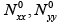and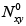). The co-ordinate system (x, y, z) chosen and the coordinate parameters are such that, the plate occupies a region given by Eq. (1).2.1 Assumptions made in theoretical formulation

Assumptions of the exponential shear deformation theory are as follows:

1. The displacements are small in comparison with the plate thickness 'h' and, therefore, strains involved are infinitesimal.

2. The in-plane displacement u in x direction as well as displacement v in y direction each consists of bending and shear componentsa) The bending componentsare assumed to be similar to the displacements given by the classical plate theory. Therefore, the expression for and can be given as.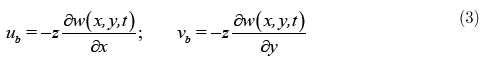b) Shear componentsare assumed to be exponential in nature with respect to thickness coordinate, such that the maximum shear stress occurs at neutral plane. Consequently, the expressions forcan be given as.where, Ф and ψ are the unknown functions associated with the shear slopes.

3. The transverse displacement w in z direction is assumed to be a function of coordinates x and y and time t.2.2 Kinematics:

Based on the above mentioned assumptions, the displacement field can be obtained using Eqs. (2) - (5) as: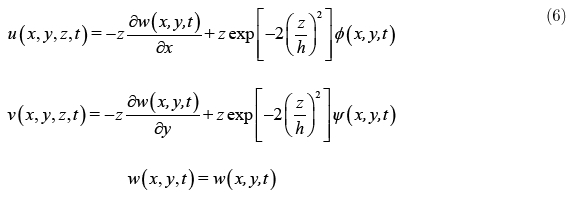This displacement field accounts for zero traction boundary conditions on the top and bottom surfaces of the plate and the parabolic variation of transverse shear strains and stresses through the thickness of plates. The kinematic relations can be obtained as follows:where,2.3 Constitutive relations

The constitutive relations for orthotropic materials are as follows:where,are the plane stress reduced elastic constants in the material axes of the plate, and are defined as:3 Derivation of Governing Equations and Boundary Conditions

The governing equations and boundary conditions are derived using principle of virtual work. Letbe the arbitrary variations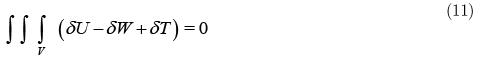where the virtual strain energy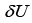virtual potential energydue to the transverse load q (x, y) and constant inplane compressive and shear forces (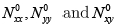) and the virtual kinetic energyare given by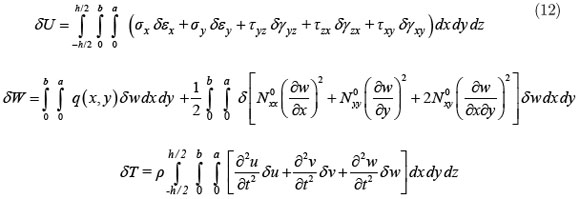Substituting Eq. (12) in Eq. (11)Substituting Eqs. (6) - (10) into the Eq. (13) and integrating through the thickness of the plate, the principle of virtual work of the plate can be rewritten aswhere, stress resultantsare defined as:Substituting Eqs. (7) and (9) into Eq. (15) and integrating through the thickness of the plate, the stress resultants are related to the generalized displacements () by the relationsVarious stiffnesses used in Eq. (16) are expressed belowIntegrating the Eq. (14) by parts, collecting the coefficients of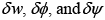the equations of motion and boundary conditions for the orthotropic plate are obtained as follows:The associated boundary conditions of a plate are as follows:Substituting Eq. (16) into Eqs. (18)-(20), the governing equations of the plate in-terms of generalized displacements are as follows: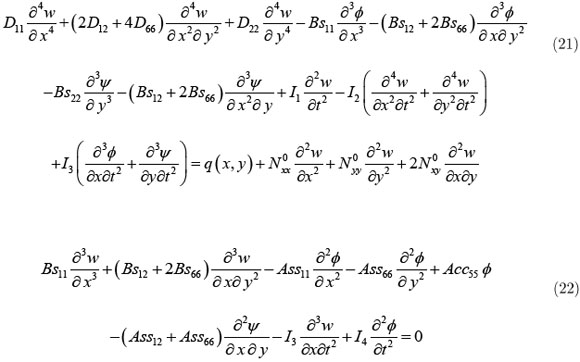4 Navier solution for simply supported square orthotropic plates

The Navier method is only applied for simply supported boundary conditions on all four edges of the rectangular plate. The following are the boundary conditions of the simply supported orthotropic plates.In order to solve the governing equations with the prescribed boundary conditions, a generalized Navier's approach is employed to obtain the closed-form solutions.

Example 1: Free vibration analysis of simply supported square orthotropic plates

The governing equations for free vibration analysis of plates can be obtained by setting the applied transverse load (q) and in-plane compressive forces (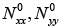and) equal to zero in Eqs. (21)-(23).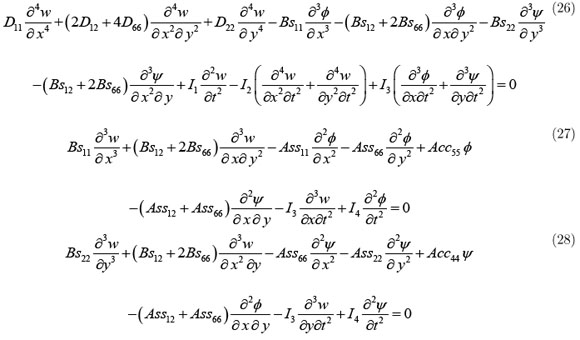The following displacement functions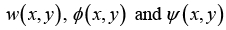are chosen to automatically satisfy boundary conditions in Eqs. (24) and (25).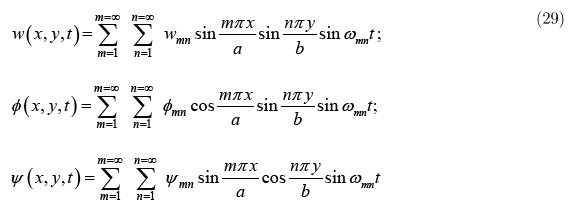whereis the amplitude of translation and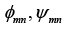are the amplitudes of rotation.is the natural frequency of mth and nth mode of vibration. Substitution of this solution form into the Eqs. (26)-(28), results in following standard Eigen value problem.where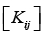is the stiffness matrix,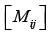is the mass matrix. Elements ofand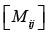are given as below:From the solution of Eq. (30), lowest natural frequency for all modes of vibration can be obtained. The orthotropic plate has following material properties.The bending mode and shear mode frequencies of orthotropic plate are presented in the following non-dimensional form.Example 2: Buckling Analysis of simply supported orthotropic plates subjected in-plane compressive forces

When a plate is subjected to in-plane compressive forces, and if the forces are small enough, the equilibrium of the plate is stable and the plate remains flat until ascertains load is reached. At that load, called the critical buckling load, the stable state of the plate is disturbed and plate seeks an alternative equilibrium configuration accompanied by a change in the load-deflection behavior. A simply supported rectangular plate subjected to the loading conditions, as shown in Fig. 1, is considered to illustrate the accuracy of the present theory in predicting the buckling behavior of the orthotropic plate

The governing equations of plate in case of static buckling are obtained by setting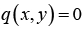,,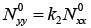and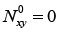in Eqs. (21) - (23).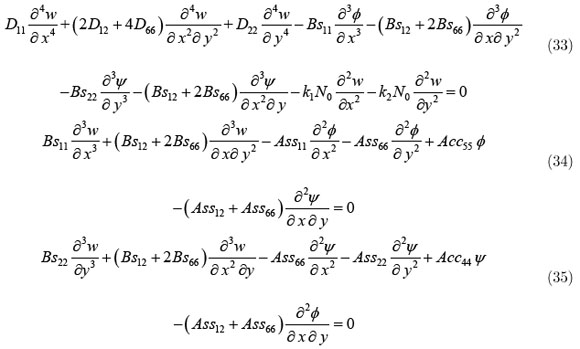The following displacement functionsare chosen to automatically satisfy the boundary conditions in Eqs. (24) and (25).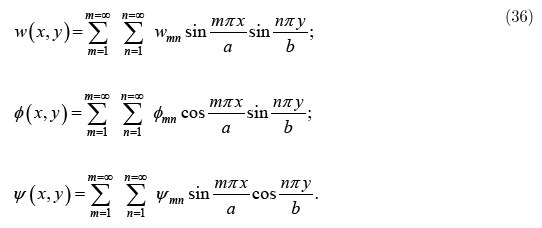Substituting Eq. (36) into Eqs. (33)-(35), the following system is obtained: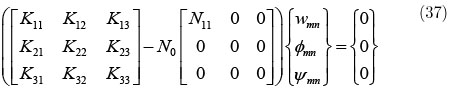where,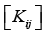are given in Eq. (31) and N11 as given below:For nontrivial solution, the determinant of the coefficient matrix in Eq. (37) must be zero. This gives the following equation for buckling load:For each choice of m and n, there is a corresponding unique value of N0. The critical buckling load is the smallest value of N0 (m, n). For verification purposes, a simply supported rectangular plate subjected to the uniaxial and biaxial loading conditions, as shown in Fig. 1, is considered to illustrate the accuracy of the present theory in predicting the buckling behavior of the orthotropic plate. The following material properties are used.Critical buckling loads are presented in the following non-dimensional form:4.2 Discussion of Results

Example 1: In the present study, free vibration analysis of an orthotropic plate with all edges simply supported is considered. Natural predominantly bending mode () and thickness shear modes (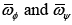) frequencies of square plate are obtained for aspect ratio 10. Non-dimensional frequencies of simply supported square plate are presented in Tables 1-3 and compared with higher order shear deformation theory (HSDT) of Reddy (1984), trigonometric shear deformation theory (TSDT) of Ghugal and Sayyad (2011b), refined plate theory (RPT) of Shimpi and Patel (2006), first order shear deformation theory (FSDT) of Mindlin (1951) and classical plate theory (CPT).

Table 1 shows comparison of predominantly bending mode frequencies of orthotropic square plate for various modes of vibration and aspect ratio 10. It is observed that the present theory yields excellent values of frequencies for all modes of vibration. The present theory and HSDT of Reddy predicts exact result of bending frequency for (m = 1, n = 1), (m = 1, n = 2), (m = 1, n = 3) and (m = 1, n = 4) modes of vibration whereas RPT and CPT overestimates the same. From Tables 2-3 it is observed that, thickness shear modes frequencies of orthotropic square plate obtained by the present theory and HSDT of Reddy are in close agreement with the exact values. The FSDT marginally overestimates the value of frequencies of this mode compared to the exact one.

Example 2: Present study also deals with the, buckling analysis of an orthotropic square and rectangular plate with all edges simply supported. Three different in-plane loading conditions are used in this numerical study: (1) uniaxial compression along the x-axis; (2) uniaxial compression along the y-axis; and (3) biaxial compression. For the comparison studies, results are also generated using higher order shear deformation theory (HSDT) of Reddy (1984), trigonometric shear deformation theory (TSDT) of Ghugal and Sayyad (2011b), first order shear deformation theory (FSDT) of Mindlin (1951) and classical plate theory.

Tables 4-5 shows the comparison of non-dimensional critical buckling load for simply supported square plate subjected to uniaxial and biaxial compression with the variation of modular and aspect ratios. It can be seen that the present theory gives excellent results of critical buckling load for all aspect ratios and modular ratio 3, whereas TSDT overestimate and FSDT underestimate the same. The differences between present theory and HSDT of Reddy will slightly increases with respect to increase in modular ratios. Classical plate theory overestimates the value of critical buckling load for all aspect ratios and modular ratios. Tables 6-8 shows the comparison of non-dimensional critical buckling load for simply supported rectangular plate. Examination of these Tables reveals that, non-dimensional critical buckling load decreases with increase in 'b/a' ratios when subjected to uniaxial compression along x-axis whereas increases when subjected to uniaxial compression along y-axis and biaxial buckling. Critical buckling load for rectangular plate increases with increase.

5 CONCLUSION

An exponential shear deformation theory (Sayyad and Ghugal, 2012a and 2012b; Sayyad, 2013) has been extended in this paper for buckling and free vibration analysis of orthotropic plates. The theory takes into account of transverse shear effects and parabolic distribution of the transverse shear strains through the thickness of the plate. From the numerical results and discussion it can be concluded that, the frequencies obtained by the present theory are accurate as seen from the comparison with exact results specially in case of natural predominately bending mode. Also, an exponential shear deformation theory can accurately predict the critical buckling loads of the orthotropic plates with various plate aspect ratios and modular ratios.

• Chen, W., Zhen, W. (2008). A selective review on recent development of displacement-based laminated plate theories. Recent Patents on Mechanical Engineering 1:29-44.
• Ghugal, Y.M., Shimpi, R.P. (2002). A review of refined shear deformation theories for isotropic and anisotropic laminated plates. Journal of Reinforced Plastics and Composites 21: 775-813.
• Ghugal, Y.M., Sayyad, A.S. (2010). A flexure of thick isotropic plate using trigonometric shear deformation theory. Journal of Solid Mechanics 2(1): 79-90.
• Ghugal, Y.M., Sayyad, A.S. (2011a).Free vibration of thick isotropic plates using trigonometric shear deformation theory. Journal of Solid Mechanics 3(2): 172-182.
• Ghugal, Y.M., Sayyad, A.S. (2011b).Free vibration analysis of thick orthotropic plates using trigonometric shear deformation theory. Latin American Journal of Solids and Structures 8: 229-243.
• Ghugal, Y.M., Pawar, M.D. (2011). Flexural analysis of thick plates by hyperbolic shear deformation theory. Journal of Experimental & Applied Mechanics 2(1):1-21.
• Karama, M., Afaq, K.S., Mistou, S. (2009). A new theory for laminated composite plates. Proceeding of Institution of Mechanical Engineers, Series L: Design and Applications 223: 53 - 62.
• Kim, S.E., Thai, H.T., Lee, J. (2009). Buckling analysis of plates using the two variable refined plate theory. Thin-Walled Structures 47: 455 - 462.
• Kreja, I. (2011). A literature review on computational models for laminated composite and sandwich panels. Central European Journal of Engineering 1(1): 59-80.
• Liew, K.M., Xiang, Y., Kitipornchai, S. (1995). Research on thick plate vibration: a literature survey. Journal of Sound and Vibration 180: 163-176.
• Mindlin, R.D. (1951). Influence of rotatory inertia and shear on flexural motions of isotropic, elastic plates. ASME Journal of Applied Mechanics 18: 31-38.
• Noor, A.K., Burton, W.S. (1989). Assessment of shear deformation theories for multilayered composite plates. Applied Mechanics Reviews 42: 1-13.
• Reddy, J.N. (1984). A simple higher order theory for laminated composite plates. ASME Journal of Applied Mechanics 51: 745-752.
• Reissner, E. (1945). The effect of transverse shear deformation on the bending of elastic plates. ASME Journal of Applied Mechanics 12(2):69-77.
• Sayyad, A.S., Ghugal, Y.M. (2012a). Bending and free vibration analysis of thick isotropic plates by using exponential shear deformation theory. Applied and Computational Mechanics 6(1): 65 - 82.
• Sayyad, A.S., Ghugal, Y.M. (2012b). Buckling analysis of thick isotropic plates by using exponential shear deformation theory. Applied and Computational Mechanics 6(2): 185 - 196.
• Sayyad, A.S. (2013).Flexure of thick orthotropic plates by exponential shear deformation theory. Latin American Journal of Solids and Structures 10: 473 - 490.
• Shimpi, R.P., Patel, H.G. (2006a). A two variable refined plate theory for orthotropic plate analysis. International Journal of Solids and Structures 43:6783-6799.
• Shimpi, R.P., Patel, H.G. (2006b). Free vibrations of plate using two variable refined plate theory. Journal of Sound and Vibration 296:979 - 999.
• Srinivas, S., Rao,C.V.J., Rao, A.K. (1970). An exact analysis for vibration of simply supported homogeneous and laminated thick rectangular plates. Journal of sound and vibration 12(2):187-199.
• Thai, H., Kim, S. (2011). Levy-type solution for buckling analysis of orthotropic plates based on two variable refined plate theory. Composite Structures 93: 1738-1746.
• Vasil'ev, V.V. (1992). The theory of thin plates.Mechanics of solids (Mekhanika Tverdogo Tela) 27:22-42, (English translation from original Russian by Allerton Press, New York)
•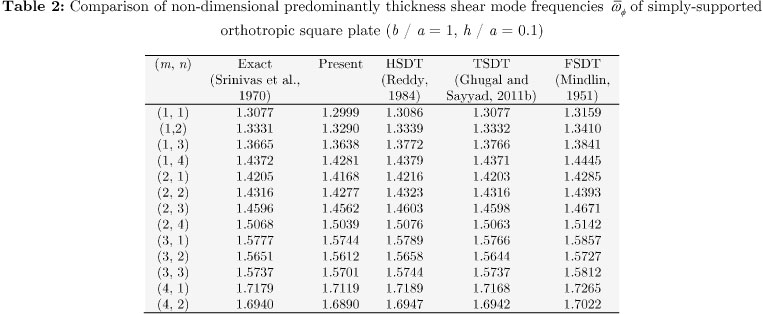•••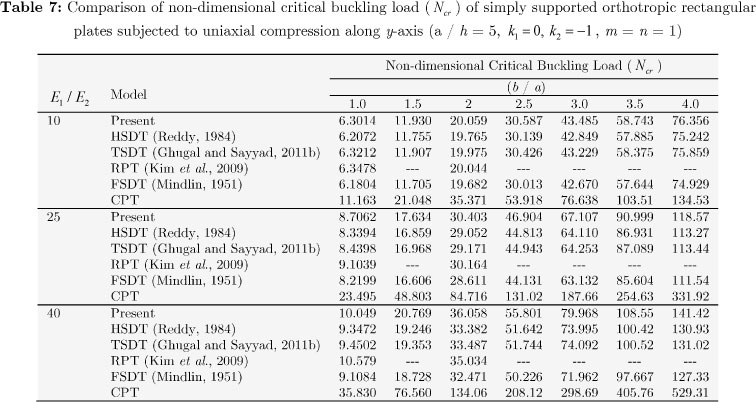•• *
Corrosponding author:

# Publication Dates

• Publication in this collection
06 May 2014
• Date of issue
Dec 2014
Associação Brasileira de Ciências Mecânicas Av. Rio Branco, 124/14º andar, 20040-001 Rio de Janeiro RJ Brasil, Tel.: (55 21) 2221 0438 - Rio de Janeiro - RJ - Brazil
E-mail: abcm@abcm.org.br